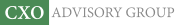Objective research to aid investing decisions

#### Value Investing Strategy (Strategy Overview)

Allocations for October 2023 (Final)
Cash TLT LQD SPY

#### Momentum Investing Strategy (Strategy Overview)

Allocations for October 2023 (Final)
1st ETF 2nd ETF 3rd ETF

# Perfect Factor Model of U.S. Stock Returns?

| | Posted in: Big Ideas, Equity Premium

How many factors are optimal for modeling future returns of individual stocks? How do these factors relate to conventionally used factors (market, size, value, momentum, investment, profitability…)? In the June 2016 version of their paper entitled “Multifactor Models and the APT: Evidence from a Broad Cross-Section of Stock Returns”, Ilan Cooper, Paulo Maio and Dennis Philip derive mathematically an optimal set of factors for predicting returns of 278 stock portfolios created by sorting U.S. stocks into tenths (deciles) according to 28 market anomalies encompassing aspects of value, momentum, investment, profitability and intangibles. They apply asymptotic principal components analysis to these portfolios to identify the factors. They quantify the premium of each of these factors as the average return spread between extreme deciles of monthly sorts of the 278 source portfolios on the factor. They then examine interactions between this mathematical factor set and several widely used empirical multi-factor models: the Fama-French 3-factor model (market, size, book-to-market); a 4-factor model (adding momentum to the 3-factor model); a second 4-factor model (adding liquidity to the 3-factor-model); a third 4-factor model (market, size, investment, profitability); and, a 5-factor model (adding investment and profitability to the 3-factor model). Using monthly returns for the 278 source stock portfolios during January 1972 through December 2013, they find that:

• There are seven mathematical factors that significantly explain the return behaviors of the 278 anomaly portfolios over the sample period.
• The first factor resembles the market factor. The other six factors capture different aspects of conventional factors (particularly value, momentum, investment and profitability).
• In aggregate, these factors explain 91% of cross-sectional return variations.
• The factors explain anomalies based on value and intangibles better than those based on investment.
• The 7-factor mathematical model explains about 60% of the cross-sectional variation of the risk premiums (differences in extreme decile returns) associated with the 28 anomalies.
• Interactions with conventional multi-factor models suggest that:
• The 7-factor mathematical model is materially superior to all widely used models.
• The 4-factor model based on market, size, investment and profitability factors is superior to the 5-factor model.
• New 7-factor empirical models selected from the 28 anomalies outperform the above widely used 3-factor, 4-factor and 5-factor models.

In summary, evidence from mathematical analysis of many anomaly portfolios indicates considerable room for improving conventional multi-factor models of U.S. stock returns via expansion to seven factors.

Cautions regarding findings include:

• All returns used are gross, not net, ignoring costs of periodic portfolio reformation based on updated sorts of stocks on factors and costs/feasibility of shorting when specified. Incorporating such costs would reduce returns, and return reductions may vary substantially across anomalies. Net (exploitable) findings may therefore differ meaningfully from gross findings.
• Cross-sectional stock return factors based on U.S. data arguably involve aggregate/cumulative snooping bias as addressed in “Taming the Factor Zoo”, thereby overstating reliability.
• The methodology employed is complex, and timely data acquisition/processing may not have been possible for much of the sample period.
• The optimal factor set is for the full sample. The optimal set may vary over time as the market evolves.
• The perfect mathematical model of U.S. stock returns may not be perfect/applicable for returns of other asset classes.